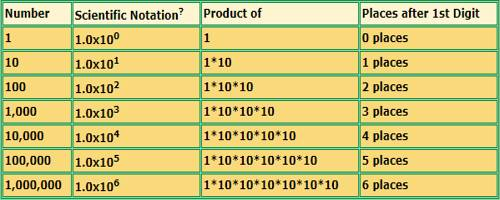Converting Numbers to Scientific Notation

Scientific notation is a standard way of writing very large and very small numbers so that they’re easier to both compare and use in computations. To write in scientific notation, follow the form

N x 10a

where N is a number between 1 and 10, but not 10 itself, and a is an integer (positive or negative number).

It is used to express very large or very small numbers. A number in scientific notation is written as the product of a number (integer or decimal) and a power of 10. This number is always 1 or more and less than 10.

For example, there are approximately 6,000,000,000 humans on earth. This number could be written in scientific notation as 6×109. The number 6,000,000,000 is equivalent to 6*1,000,000,000. The number 1,000,000,000 is equivalent to 109 or 10*10*10*10*10*10*10*10*10.A number can be converted to scientific notation by increasing the power of ten by one for each place the decimal point is moved to the left. In the example above, the decimal point was moved 9 places to the left to form a number more than 1 and less than 10.

Scientific notation numbers may be written in different forms. The number 6×109 could also be written as 6e+9. The +9 indicates that the decimal point would be moved 9 places to the right to write the number in standard form.

Convert from Real Number to Scientific Notation:

0.000345 = 3.45 × 10-4

Here we wish to write the number 0.000345 as a coefficient times 10 raised to an exponent. To convert to scientific notation, start by moving the decimal place in the number until you have a coefficient between 1 and 10; here it is 3.45. The number of places to the left that you had to move the decimal point is the exponent. Here, we had to move the decimal 4 places to the right, so the exponent is -4.

0.000345

= 0.00345 / 10

= 0.0345 / (10 × 10)

= 0.345 / (10 × 10 × 10)

= 3.45 / (10 × 10 × 10 × 10)

= 3.45 / (104)

= 3.45 × 10-4

Information Source: